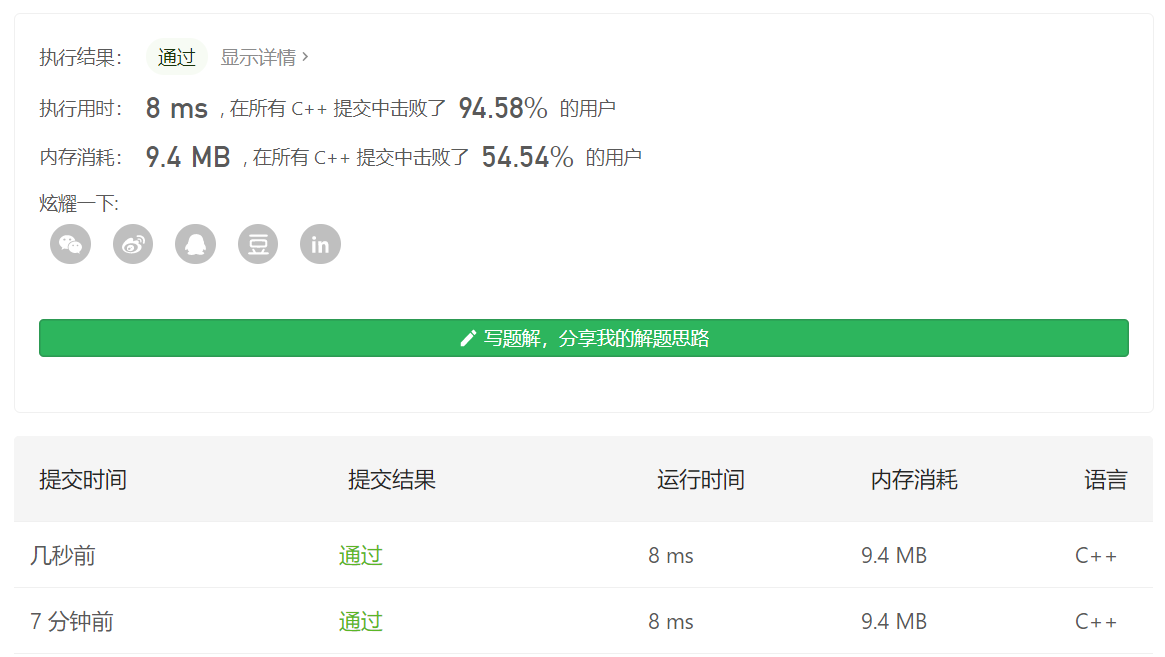# liyirui's Blog

##### Leetcode-1239. 串联字符串的最大长度``````// 本题的最优解的最大值就是26，也就是说，DP数组其中一个维度的最大值就是26
// 字符串中最多也只出现26个字母，于是可以用32位的int来存储
class Solution {
private:
const int Size = 26;
public:
int countOne(int a){
int ct = 0;
while(a != 0){
a = a & (a-1);
ct++;
}
return ct;
}

int maxLength(vector<string>& arr) {
int size = arr.size();
vector<int> newarr;
// 将字符串存储转换为int其实只用到26位
for(int i = 0; i < size; i++){
int val = 0;
for(char c : arr[i]){
int bit = 1 << (c - 'a');
// (val & bit) == 0 说明这个字母在这个单词中目前没有出现过
if((val & bit) == 0){
val += bit;
}
// 如果这个字母在这个单词中已经出现过，这个就没用了
else{
val = 0;
break;
}
}
if(val != 0) newarr.push_back(val);
}

// 建立dp数组
vector<vector<int>> dp (Size);
for(int i = 0; i < newarr.size(); i++){
int ct = countOne(newarr[i]);
// 先看看可不可以将自己加在别的字符串后面
for(int j = Size-1; j >= 0; j--){
for(int k = 0; k < dp[j].size(); k++){
if((newarr[i] & dp[j][k]) == 0){
dp[j+ct].push_back(newarr[i]+dp[j][k]);
}
}
}
// 再把自己插进去
dp[ct-1].push_back(newarr[i]);
}

for(int i = Size-1; i >= 0; i--){
if(dp[i].size() >= 1) return i+1;
}
return 0;
}
};
``````

### 回文串系列-Manacher算法

##### Leetcode-5. 最长回文子串（Manacher算法）

1. 先把字符串的每个字符之间插入一个 `#` 生成新的字符串，这样做的好处是，避免了分情况讨论奇数长度的回文串和偶数长度的回文串，转换完都变成了奇数长度的回文串。例如，`abbas` 转换成 `#a#b#b#a#s#`

2. 设置三个变量：

• ptr：记录遍历输入字符串所到达的最远的地方，ptr-1是以index为中心的回文串所能到达的最右的位置，ptr的位置被验证过，不满足回文的条件。ptr保证了不回头遍历，也就保证了\$O(n)\$ 的时间复杂度。
• index：记录的是得到当前的ptr所对应的回文串的中心。
• vec[]：vec[i]表示以i为中心的回文串的最大半径（假设回文串的长度为n，那么半径 r = (n+1)/2 ）。
3. 开始更新vec，此时设置一个i，沿着字符串从左向右递增。ptr初始化为0，index初始化为0，i初始化为0，vec初始化为全0。

• 如果 \$ptr \le i\$ ，也就意味着i这一位置未曾遍历过，所以此时以i为中心，向左向右扩展，找到以i为中心所能得到的回文串的最左和最右的边界。随着向右的扩展，ptr也一直变大，直到在某一个位置处，不再满足回文。此时以i为中心的回文串的最右边界为`ptr-1`。从而，`vec[i] = ptr-i`，ptr改变，index也要相应改变，这里index同时更新为i的值。

• 如果\$ptr > i\$，也就意味着i这个位置之前已经被遍历过，所以可以利用之前遍历得到的记录，来得到vec[i]的值。这里主要借助的是对称性，找到`i`关于`index`对称的的点`i'`。这里又分成三种情况：

这里，将以index为中心的回文串的最左端叫做index最左，最右端叫做index最右，也就是ptr-1

• 通过`i'`知道，以`i'`为中心的回文串最左也在index最左之内，所以以`i`为中心的回文子串的最右也在index最右内。那么根据对称性，以`i'`为中心的字符串的长度，也是以`i`为半径的字符串的长度。
• 通过`i'`知道，以`i'`为中心的回文串最左刚好是index最左，此时可以知道以`i`为中心的字符串最右至少是到`ptr-1`，后面的需要利用左右扩展去验证。在验证的同时`ptr`也随之扩展，同时别忘了更新`index`
• 通过`i'`知道，以`i'`为中心的回文串最左在index最左之外，那么`i` 的最右刚好就是`ptr-1`，假如`ptr`也包含在以`i`为中心的字符串内，那么，根据对称性，index的最左又要往左移动一个，这是矛盾的。
4. 最后，遍历一遍vec，找到其中半径的最大值，将以该点为中心的字符串去掉`#`输出。

``````class Solution {
private:
// 给字符串中插入#
string manacherStr(string &str){
string new_str = "";
for(char c : str){
new_str.append(1, '#');
new_str.append(1, c);
}
new_str.append(1, '#');
return new_str;
}
// 计算从ptr开始，有多少对以index为中心的字符，遇到第一个不一样的便停止
int palindromeCount(string &str, int center, int &ptr){
int count = 0;
int _ptr = (center << 1) - ptr;
while(_ptr >= 0 && ptr < str.size() && str[_ptr] == str[ptr]){
count++;
_ptr--;
ptr++;
}
return count;
}
// manacher算法
vector<int> manacher(string &s){
s = manacherStr(s);
vector<int> vec (s.size(), 0);
int ptr = 0, index = 0;
for(int i = 0; i < s.size(); i++){
if(ptr <= i){
vec[i] = palindromeCount(s, i, ptr);
index = i;
}
else{
int _i = (index << 1) - i;
if(i + _radius - 1 < ptr-1){
}
else if(i + _radius - 1 > ptr-1){
vec[i] = ptr - i;
}
else{   //i + _radius - 1 == ptr - 1
vec[i] = ptr - i;
vec[i] += palindromeCount(s, i, ptr);
index = i;
}
}
}
return vec;
}
public:
string longestPalindrome(string s) {
vector<int> vec = manacher(s);
string ans = "";

// 寻找最长回文串
int maxi = 0, max = vec;
for(int i = 0; i < vec.size(); i++){
if(vec[i] > max){
max = vec[i];
maxi = i;
}
}

// 解码输出
int offset = maxi - max + 1, len = (max << 1) - 1;
for(int i = 0; i < len; i++){
if(s[offset+i] != '#'){
ans.append(1, s[offset+i]);
}
}

return ans;
}
};
``````

### KMP算法

KMP算法解决上面这个问题的时间复杂度为O(M+N)，这里haystack的长度为M，needle的时间复杂度为N。

KMP算法的核心思想是，haystack上的指针不回头。在一处匹配失败以后，needle的匹配结果，可以作为指导needle上的指针跳转位置的依据。这个需要提前算出来。